×

Search anything:

# Check whether a Singly Linked List is Palindrome or not

#### Algorithms Data Structures linked listA number is Palindrome if it reads same from front as well as back.For example,
2332 is palindrom number as its read same from both sides.

Linked List can also be palindrome if they have the same order when it traverse from forward as well as backward.

It can be done with using three different methods:

• Using stack
• Using string
• By reversing the list

## Using Stack:

### Algorithm

• Traverse the linked list and push the value in Stack.
• Now, again traverse the linked list and while doing so pop the values from the stack.If stack become empty it means given Linked list is pallindrom otherwise not.

Given below is a Singly Linked List: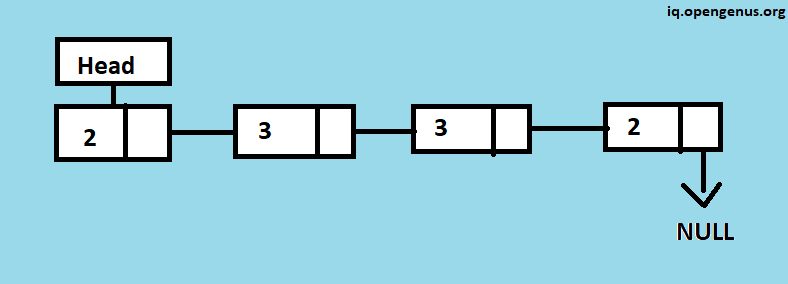### Step 1: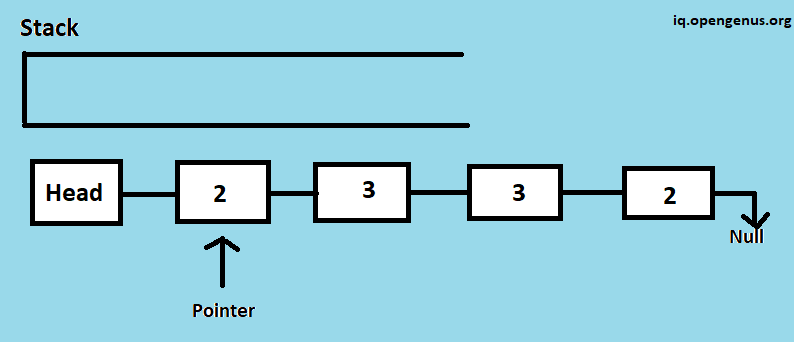Currently,pointer is at the beginning of the list which points to Node value 2

### Step 2:Now,After the Node 2 is visited pointer moves forward and the visited node will be push into the stack.

### Step 3: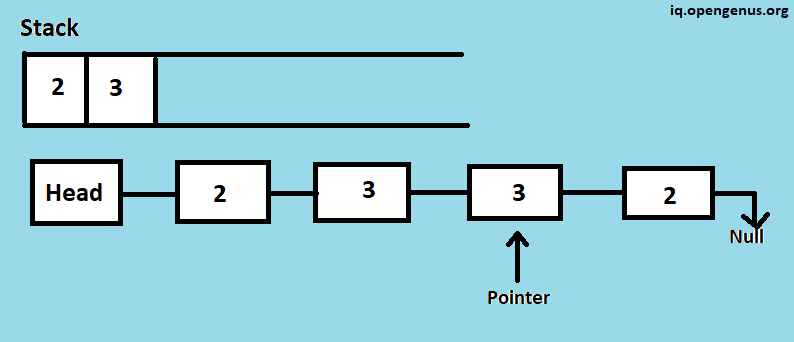Node 3 is visited,push Node 3 into Stack and moves the pointer forward.

### Step 4: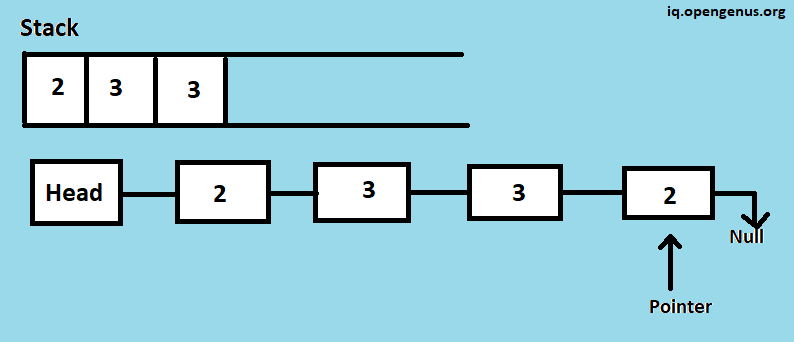Node 3 is visited,push Node 3 into Stack and moves the pointer forward.

### Step 5: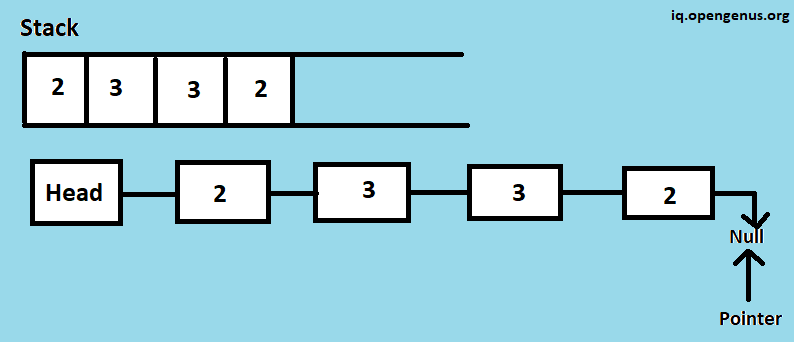Node 2 is visited,push Node 2 into Stack and moves the pointer forward until it's NULL.

### Step 6: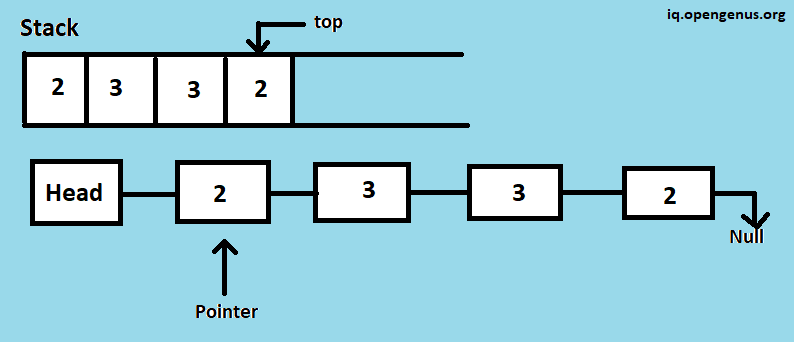Now,our approach will be to traverse the list once again from the beginning and this time will be ke checking is the Stack.top() is equal to the current node or not.Here,top value of stack is 2 and current node value of list is 2 they both are equal then pop the top value from the stack and move the pointer forward,repeat these steps until list found to be NULL.
Whenever you found that top value of stack is not equal to the current value of node then return false.

### Step 7: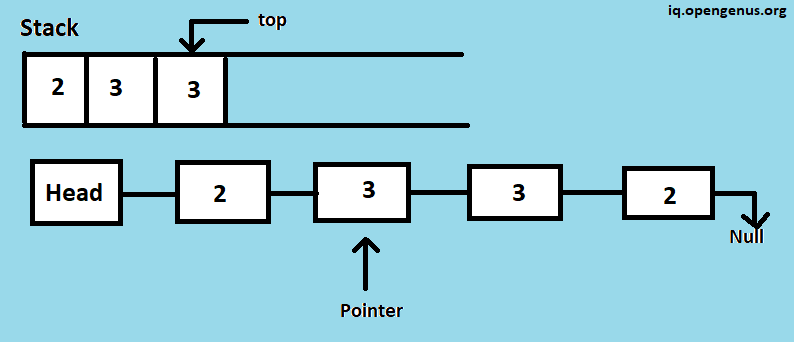### Step 8: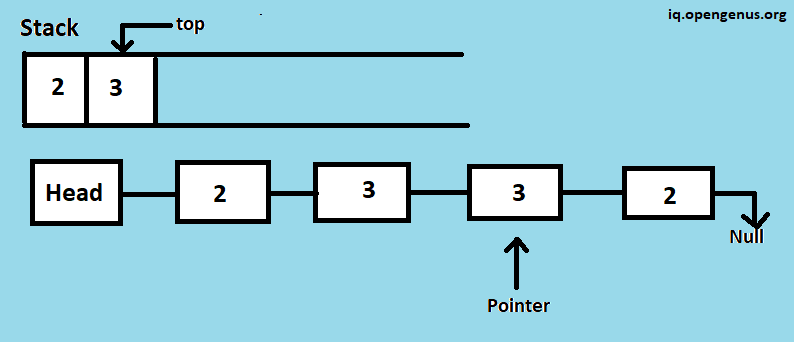### Step 9: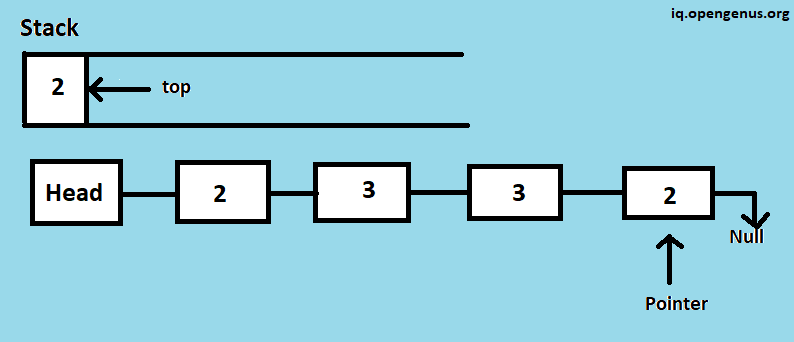### Step 10: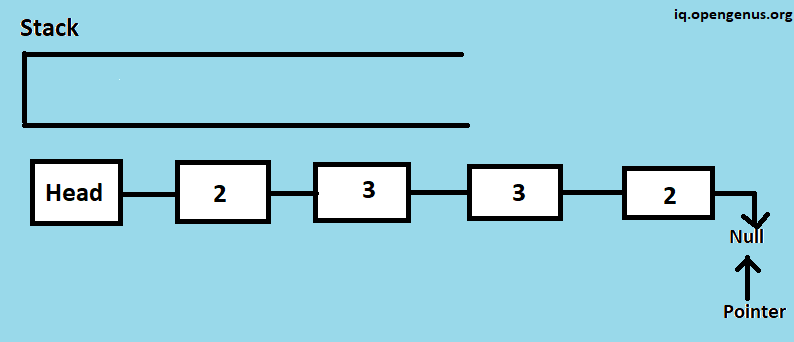### The following code implements the above algorithm:

``````#include<bits/stdc++.h>
using namespace std;

typedef struct Node {
int data;
struct Node * next;
} Node;

Node * createNode(int val){
Node * temp = (Node *)malloc(sizeof(Node));
if(temp){
temp->data = val;
temp->next = NULL;
}
return temp;
}

/* This function inserts node at the head of linked list */
Node * newNode  = createNode(data);
}

}
cout<<"Null";
}
{

//Declare a Stack
stack<int>s;

//Push all the values of list into the stack
while(temp)
{
s.push(temp->data);
temp=temp->next;
}

// Get the top most element
int val=s.top();

// Pop the element
s.pop();

//Check is the data in the stack and list is same or not
return false;
}

}
return true;
}
int main()
{
cout<<"Original list :";
//function to Check list is Palindrome or not
else cout<<"\nGiven List is not Palindrome";
}
``````

## Another method using String:

Idea: Add List values to a string and then check if reverse of that string become equal to the original string then it is palindrome otherwise not.

``````#include<bits/stdc++.h>
using namespace std;

string check;

typedef struct Node {
int data;
struct Node * next;
} Node;

Node * createNode(int val){
Node * temp = (Node *)malloc(sizeof(Node));
if(temp){
temp->data = val;
temp->next = NULL;
}
return temp;
}

/* This function inserts node at the head of linked list */
Node * newNode  = createNode(data);
}

}
cout<<"Null";
}
int main()
{
cout<<"Original list :";
//fCheck list is Palindrome or not
string val=check; reverse(val.begin(),val.end());
if(check==val)cout<<"\nGiven List is Palindrome";
else cout<<"\nGiven List is not Palindrome";
}
``````

### Other Approaches:

#### By Reversing the List:

This method takes O(n) time complexity and O(1) extra space.
Approach of this method is to find the middle element of linked list,then take second half list and reverse it,compare this with first half of list if they become equal it means List is pallindrome otherwise not.

#### Approach:-

For getting the middle element of list take two pointer one is start and other is end,move pointer start by one and pointer end by two.When end pointer reached last node start pointer will be at the middle.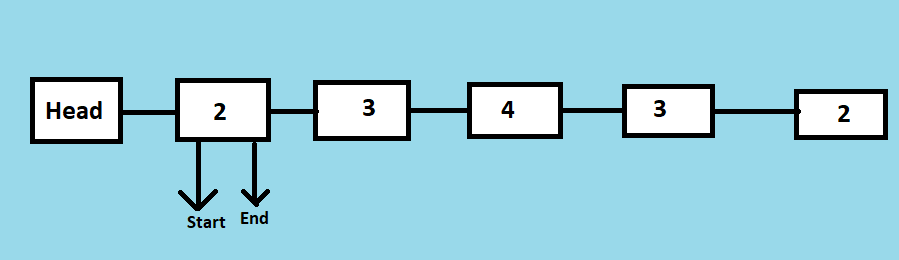Here moving start pointer by one and end pointer by two: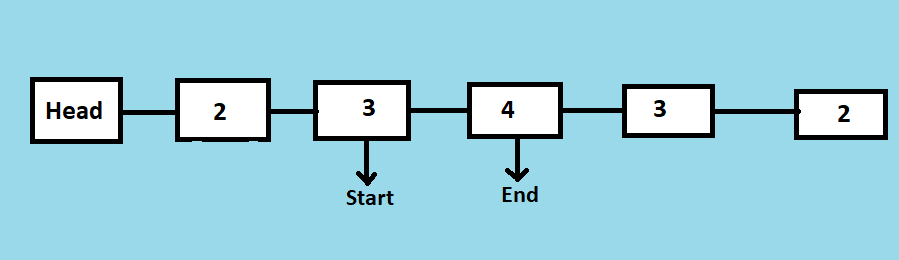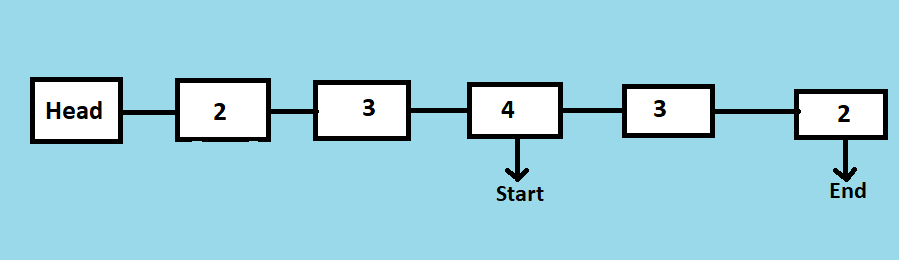Here,finally start pointer is pointing to the middle element of the list.Our next task will be to reverse the second half of list and then compare from the first list.

### Pseudocode:

For finding the middle element of the list and reversing the second half of list:-

``````bool PalindromeCheck(struct Node* head)
{
struct Node *second_half, *prev_start = head;
struct Node* midnode = NULL; // case when list have odd number of size
bool result = true; // initialize result

//Getting the middle of the list by moving start pointer 1 and end pointer by 2
while (end != NULL && end->next != NULL) {
end = end->next->next;

/*We need previous of the start_ptr for
linked lists with odd elements */
prev_start = start;
start = start->next;
}

if (end != NULL) {
midnode = start;
start = start->next;
}

// Now reverse the second half and compare it with first half
second_half = start;
prev_start->next = NULL; // NULL terminate first half
reverse(&second_half); // Reverse the second half
result = compare(head, second_half); // compare

}
return res;
}
``````

Compairing first half and second half of list

``````bool compare(struct Node* head_of_first, struct Node* head_of_second)
{

while (temp1 && temp2) {
if (temp1->data == temp2->data) {
temp1 = temp1->next;
temp2 = temp2->next;
}
else
return false;
}

// If Both are empty reurn 1
if (temp1 == NULL && temp2 == NULL)
return true;

//It means one of list become NULL so given list is not pallindrome
return false;
}
``````

Some other methods are also there like by using recursion,you can give it a try to do this.